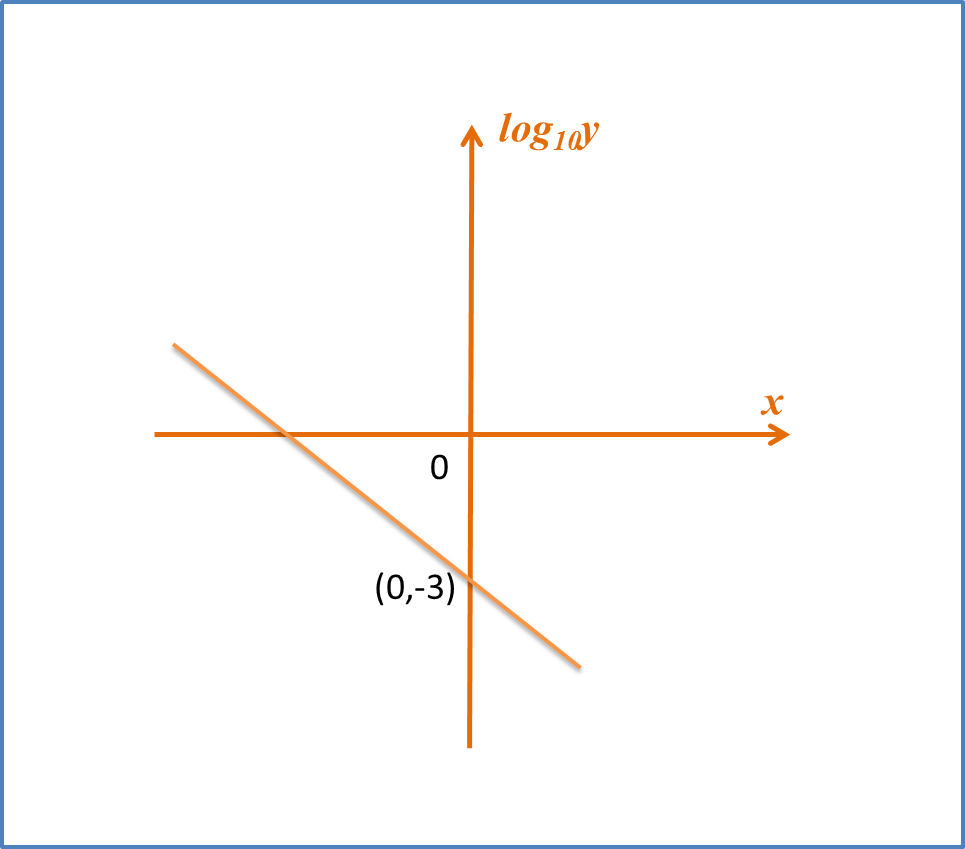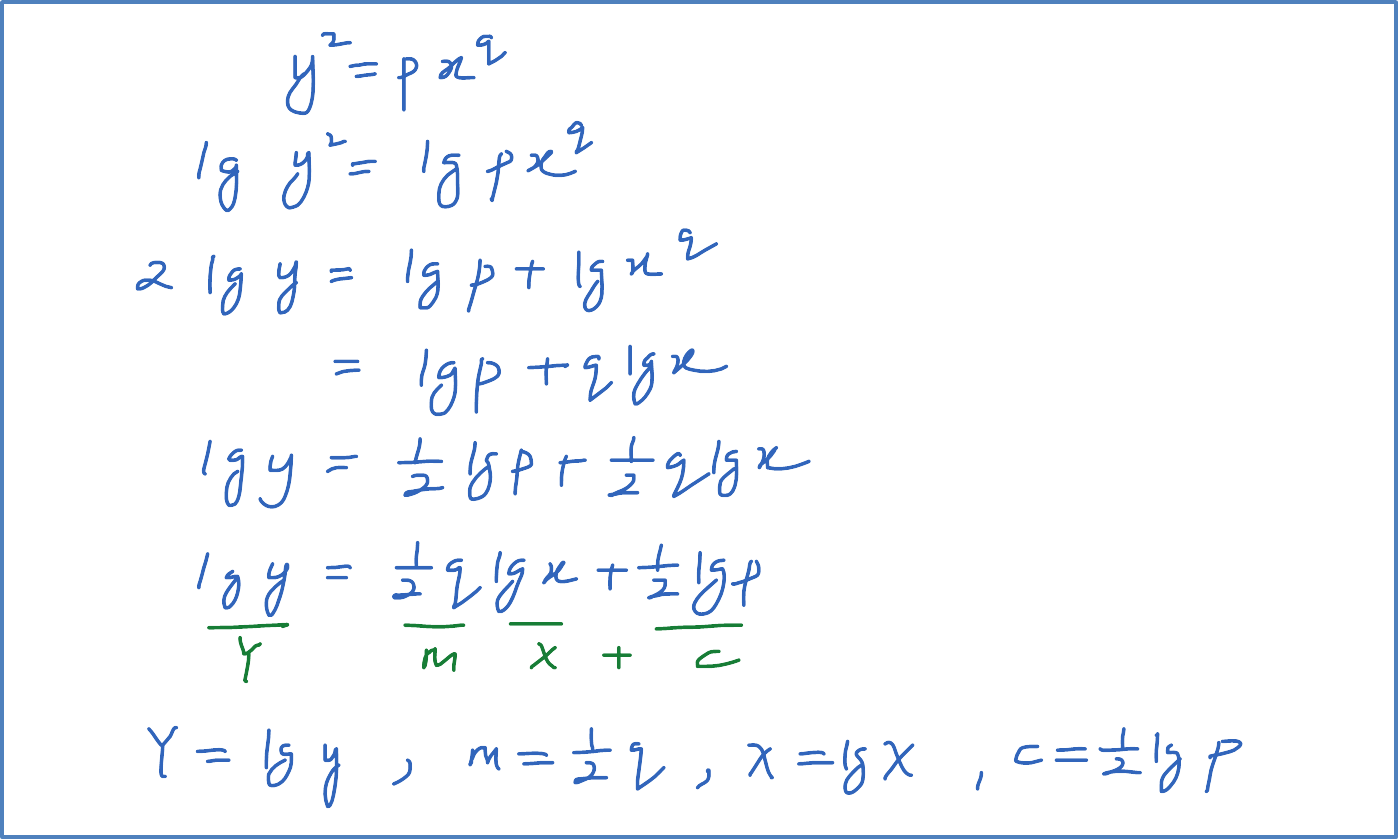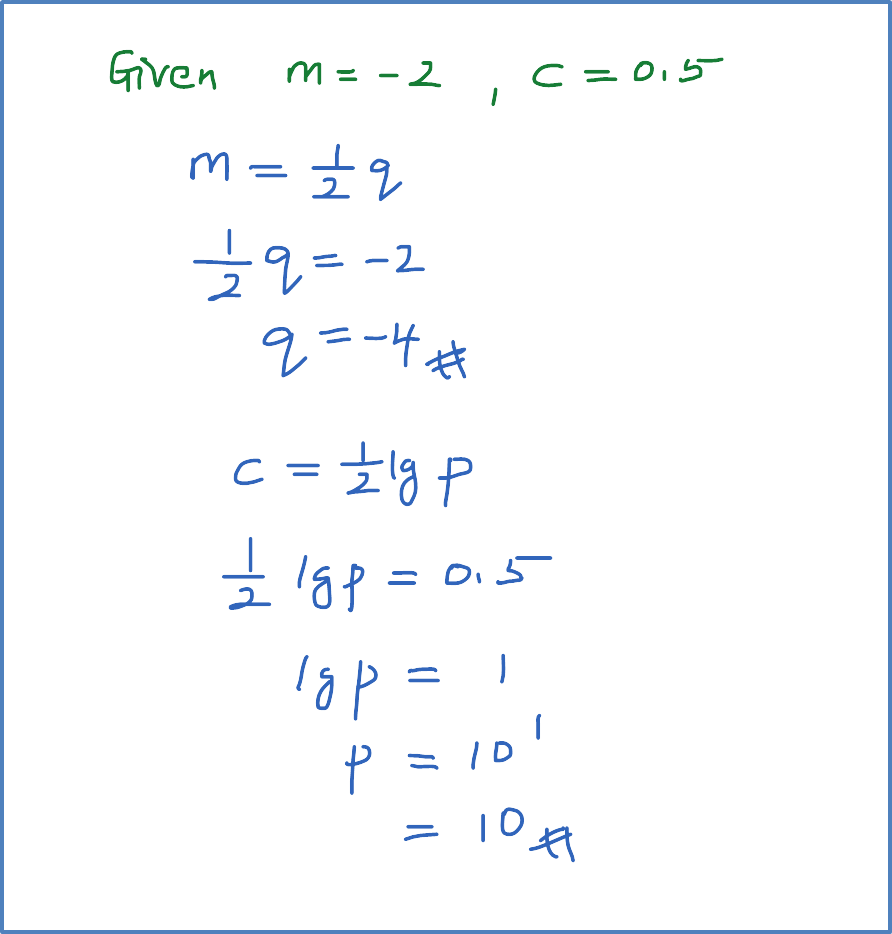# SPM Practice 2 (Question 8 – 10)

Question 8:
The variables x and y are related by the equation $y=\frac{p}{{3}^{x}}$, where k is a constant.
Diagram below shows the straight line graph obtained by plotting ${\mathrm{log}}_{10}y$ against x.1. Express the equation$y=\frac{p}{{3}^{x}}$ in its linear form used to obtain the straight line graph shown in Diagram above.
2. Find the value of p.

Solution:Question 9:
Variable x and y are related by the equation ${y}^{2}=p{x}^{q}$ . When the graph lg y against lg x is drawn, the resulting straight line has a gradient of -2 and an vertical intercept of 0.5 . Calculate the value of p and of q.

Solution:Question 10:
Variable x and y are related by the equation $y=\frac{c}{d-x}$. When the graph y against xy is drawn the resulting line has gradient 0.25 and an intercept on the y-axis of 1.25. Calculate the value of c and of d.

Solution: